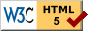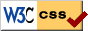# WeBWorK Story Problem Maker

When creating online homework for my undergraduate reals class, I needed to create a lot of homeworks that were mostly just draggable proofs, radio boxes, some simple number/formula entry, and checkboxes, with minimal to no setup. Doing cut and paste with the WeBWorK code was taking up way too much time, so I created a new syntax. The original idea was for longer problems, with test questions interspersed, but it is usable for all sorts of simple questions. See below the textbox for a (long) sample file.

Enter the problem into the textbox below and press "Compile".

## Sample File / Syntax

Here is a sample file, that also explains the syntax.

This is an example story proof thingie with interspersed questions.  Here's
some math $$\int_0^1 f(x)\,dx .$$  We can have proofs, radio boxes, check
boxes, numbers and formulas, including some simple setup.

That was a paragraph break (we left an empty line).  Leaving two empty lines
gives two paragraph breaks (very large break).  If you want to just make the
file more readable you can include % on a line by itself and that just does
nothing (no new paragraph break).
%
The rest of the syntax is like regular WeBWorK syntax (i.e. not PGML).  Normal
text just gets copied verbatim into the problem file.  So you have to follow
WeBWorK idiosyncracies such as $$LB 1,2,3 RB$$ for the braces as
slash-brace has a meaning in WeBWorK.  You can also do some styling with the
standard WeBWorK styling with variables.  The next line is bold.

$BBOLD Proofs$EBOLD

Proofs require draggableProof.pl to work (presumably from WeBWorK 2.16
onwards this works automatically).

%PROOF
First statement
Second statement
Third statement
%EXTRA
Extra wrong statement
Another Extra wrong statement
%END

We can also have another proof surely, maybe without any wrong statements

%PROOF
Statement $$x \not= y$$
Another Statement
%END

And we can add a hint on the number of correct statements

%PROOFHINT
Statement $$x \not= y$$
Another Statement
%EXTRA
Wrong statement
%END

$BBOLD Radio buttons$EBOLD

There are also radio buttons (here with only a linebreak).
$BR %RADIO Wrong 1 (some math $$x^2$$) Wrong 2 %CORRECT Correct Wrong 3 %END And another radio thing.$BR
True
%CORRECT False
%END

Here are radio buttons randomized except for the last option which always comes
last.  And there's a whole paragraph break.

%BEGINRANDOM
%CORRECT Choice A
Choice B
Choice C
%ENDRANDOM
None of these
%END

There is a short version of this since I'm lazy:

%CORRECT Choice A
Choice B
Choice C
%ENDRANDOM
None of these
%END

The %ENDRANDOM is added automatically if needed, so if we want to just
randomize everything we can write:

%CORRECT Choice A
Choice B
Choice C
%END

The given first option is taken as the correct answer in case nothing is marked
as correct, so the following is the same as above, just a bit less readable.

Choice A
Choice B
Choice C
%END

$BBOLD Check all that applies (check boxes)$EBOLD

There are also check-all-that-applies type things, always randomized.  First
come the correct ones, then the extras, you can make one specific thing be
always last, otherwise they are all randomized.

%CHECKBOXES
Correct 1
Correct 2
%EXTRA
Incorrect 1
%LAST None of the above
%END

%CHECKBOXES
Correct 1
%LAST Correct 2 and always last
%EXTRA
Incorrect 1
%END

(Note that checkboxes automatically adds a line break in front, so unlike
for radio buttons you can just start CHECKBOXES on the next line)
%CHECKBOXES
Correct 1
Correct 2
Correct 3
%END

For radio buttons and checkboxes, if you want to avoid giving hints after
of a line.  If you want to give all or nothing rather than partial credit,
put %NOPARTIALCREDIT at the beginning of a line.  Presumably you'd use the
first few lines of the file for these.  You can also just use %NOPARTIAL to
turn on both of these, which is the most common option

$BBOLD Inputs (formulas, numbers, strings)$EBOLD

If we want to have formulas, add %FORMULAVARS x,y on a separate line
where the list is a comma separated (no spaces) list of variable names
all formulas here will use.

%FORMULAVARS x,y,z

more complicated setup).

%FORMULA x^2+y^2+z^2

We can also ask for a number.  By the way, note how the answer blank
comes on the same line.  You'd need to add a line break if you want it to
come on the next line.

$BR %NUMBER 42 We can randomize by adding some setup and using that. Note that setup is always put at the top of the output file even though you can specify it wherever. But it does come in the right order. It can really be kind of anything. %SETUP$a=random(2,9,1);
Note that you can also use %S as shorthand for %SETUP.

What's 2 times $a? %NUMBER 2*$a

Normally the answer rule width is 20 for numbers and 40 for formulas.  With
the %RULEWIDTH statement you can change it to another width (used for all
rules from then on.)  The next rule will be 80-wide.

%RULEWIDTH 80
%NUMBER 42

If you want to go back to the defaults just set rule width to 0.

%RULEWIDTH 0

Now the number field will be again 20-wide.

%NUMBER 42

We can also have a string.  Normally whitespace and case is more or less
ignored, as long as the same words are given so "good bye" and "  Good   BYE "
would be equivalent, but "goodbye" is not.  By default it is a 40-wide rule.

%STRING Good Bye

You can also ask for matrices.  If any matrix entry is encountered, the
MathObjects context is changed to "Matrix" for the whole problem.
The default rule width for matrices is 10 because this will be for every
entry.

%MATRIX [[1,2],[3,4]]

That asks for matrices by giving an entry array, but sometimes you want
to see if the student knows what size the matrix should be, so you just
want to give one entry where the matrix has to be entered using the webwork
syntax.  For that use MATRIXENTRY.  Default rule width is 40.

%MATRIXENTRY [[1,2],[3,4]]

$BBOLD Advanced variable setup$EBOLD

If a range of values is to be used for a variable when testing formulas you can
put %VARRANGE x [2,5] on a separate line.  So we will test formulas with x in
that range.  It is equivalent to
adding "%SETUP Context()->variables->set(x => {limits => [2,5]});" on a line,
but is a little bit faster to type.  If you have other setup before, this can
obviously include variables such as [$a,$b] or something like that.

%VARRANGE x [2,5]

Note that by default the context is set up with the flags
num_points=50, granularity=10000, tolType="relative", tolerance=0.00001,
reduceConstants=0, reduceConstantFunctions=0, which is a rather stricter than
the normal webwork setup which is kind of loose.  You can change it by
adding %SETUP flags of course, or modifying the output.  The variables
from %FORMULAVARS are by default set to "Real" type.

$BBOLD Descriptive headers$EBOLD

You can also include the standard description headers by "%H text here".
The "%DESC Description here" line would expand to the BEGINDESCRIPTION /
ENDDESCRIPTION comment.  Headers are put at the very very top of the output no
matter where they are in your input.

The "%DESC" line shuold be just one line (otherwise you should just use %H).
This line will also be added into a "COMMENT();" statement that is visible
in the Library Browser in WeBWorK.  Alternatively you can add a line
with "%COMMENT Comment here":
%COMMENT This is a comment for this file.

$BBOLD Large fonts$EBOLD

Sometimes font is too small in the HTML to be readable, so for some problems
it's better to just automatically zoom things.  Adding "%LARGEFONT" on an empty
line towards the very beginning of the file will increase font size in the
stylesheets to 120%.  "%HUGEFONT" will make it 150%.  This only affects the HTML
and doesn't do anything to the hardcopy versions.

$BBOLD Solutions$EBOLD

Finally a solution can be started by putting %SOLUTION on an empty line.
Everything after that will be in the solution.
%
%
%SOLUTION
This text is in the solution.  Once you start the solution, that's it,
everything after is in the solution.

Same exact rules for empty lines causing paragraph breaks.  However, none of
the question things work, of course, since you can't ask questions in the
solution.


## Where can I find this script?

See my github repo for various webwork related stuff, mostly problems I wrote. The script is the story-proof-template-maker.pl perl script. It is rather rudimentary and doesn't have much in terms of handling errors. It just gives bad output usually when the input is not correct syntax.